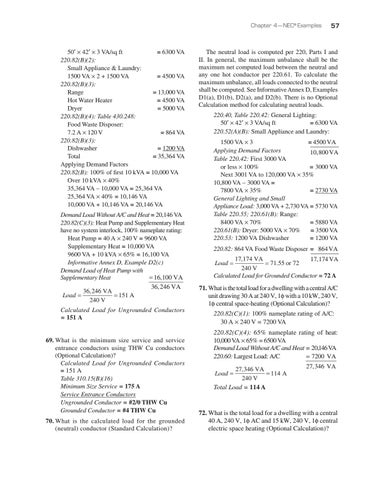Chapter 4 — NEC® Examples

50′ × 42′ × 3 VA/sq ft = 6300 VA 220.82(B)(2): Small Appliance & Laundry: 1500 VA × 2 + 1500 VA = 4500 VA 220.82(B)(3): Range = 13,000 VA Hot Water Heater = 4500 VA Dryer = 5000 VA 220.82(B)(4): Table 430.248: Food Waste Disposer: 7.2 A × 120 V = 864 VA 220.82(B)(3): Dishwasher = 1200 VA Total = 35,364 VA Applying Demand Factors 220.82(B): 100% of first 10 kVA = 10,000 VA Over 10 kVA × 40% 35,364 VA – 10,000 VA = 25,364 VA 25,364 VA × 40% = 10,146 VA 10,000 VA + 10,146 VA = 20,146 VA Demand Load Without A/C and Heat = 20,146 VA 220.82(C)(3): Heat Pump and Supplementary Heat have no system interlock, 100% nameplate rating: Heat Pump = 40 A × 240 V = 9600 VA Supplementary Heat = 10,000 VA 9600 VA + 10 kVA × 65% = 16,100 VA Informative Annex D, Example D2(c) Demand Load of Heat Pump with Supplementary Heat = 16,100 VA 36, 246 VA 36, 246 VA Load = = 151 A 240 V Calculated Load for Ungrounded Conductors = 151 A 69. What is the minimum size service and service entrance conductors using THW Cu conductors (Optional Calculation)? Calculated Load for Ungrounded Conductors = 151 A Table 310.15(B)(16) Minimum Size Service = 175 A Service Entrance Conductors Ungrounded Conductor = #2/0 THW Cu Grounded Conductor = #4 THW Cu 70. What is the calculated load for the grounded (neutral) conductor (Standard Calculation)?

57

The neutral load is computed per 220, Parts I and II. In general, the maximum unbalance shall be the maximum net computed load between the neutral and any one hot conductor per 220.61. To calculate the maximum unbalance, all loads connected to the neutral shall be computed. See Informative Annex D, Examples D1(a), D1(b), D2(a), and D2(b). There is no Optional Calculation method for calculating neutral loads. 220.40, Table 220.42: General Lighting: 50′ × 42′ × 3 VA/sq ft = 6300 VA 220.52(A)(B): Small Appliance and Laundry: 1500 VA × 3 = 4500 VA Applying Demand Factors 10, 800 VA Table 220.42: First 3000 VA or less × 100% = 3000 VA Next 3001 VA to 120,000 VA × 35% 10,800 VA – 3000 VA = 7800 VA × 35% = 2730 VA General Lighting and Small Appliance Load: 3,000 VA + 2,730 VA = 5730 VA Table 220.55; 220.61(B): Range: 8400 VA × 70% = 5880 VA 220.61(B): Dryer: 5000 VA × 70% = 3500 VA 220.53: 1200 VA Dishwasher = 1200 VA 220.82: 864 V VA Food Waste Disposer = 864 VA 17,174 VA 17, 174 VA = 71.55 or 72 240 V Calculated Load for Grounded Conductor = 72 A Load =

71. What is the total load for a dwelling with a central A/C unit drawing 30 A at 240 V, 1φ with a 10 kW, 240 V, 1φ central space-heating (Optional Calculation)? 220.82(C)(1): 100% nameplate rating of A/C: 30 A × 240 V = 7200 VA 220.82(C)(4): 65% nameplate rating of heat: 10,000 VA × 65% = 6500 VA Demand Load Without A/C and Heat = 20,146 VA = 7200 VA 220.60: Largest Load: A/C 27, 346 VA = 114 A 240 V Total Load = 114 A Load =

27, 346 VA

72. What is the total load for a dwelling with a central 40 A, 240 V, 1φ AC and 15 kW, 240 V, 1φ central electric space heating (Optional Calculation)?

Journeyman Electrician's Exam Workbook Based on the 2017 NEC®

Journeyman Electrician’s Exam Workbook Based on the 2017 NEC® is designed to help applicants prepare for the journeyman electrician’s state...

Journeyman Electrician's Exam Workbook Based on the 2017 NEC®

Journeyman Electrician’s Exam Workbook Based on the 2017 NEC® is designed to help applicants prepare for the journeyman electrician’s state...